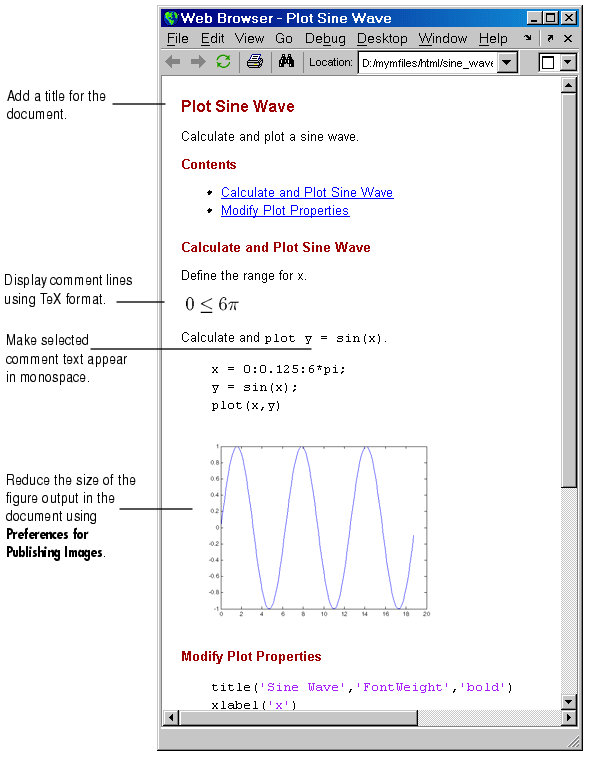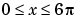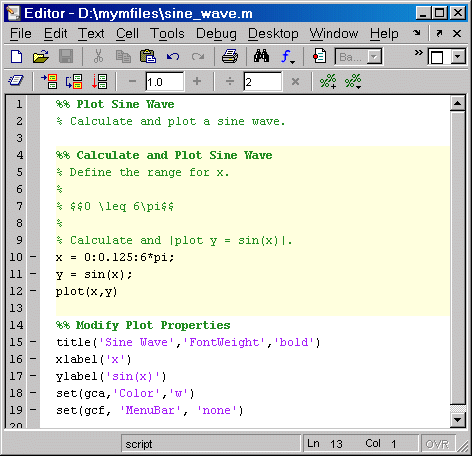Desktop Tools and Development EnvironmentExample of Publishing with Text Markup

This simple example adds text markup to the sine_wave.m file used in Example of Publishing Without Text Markup to produce the following published HTML document. General instructions for marking up M-files for publishing are in the section after the following example.1. Add an overall title for the published document
1. Add a blank line at the top of the file.
2. Select Cell -> Insert Text Markup -> Cell Title. MATLAB adds the following in the new blank line and adds a blank line beneath it.
• %% TITLE

The %% indicates the start of a new cell, where a cell is a section of an M-file.

1. Type over the text TITLE, replacing it with Plot Sine Wave. Add a comment about the overall file in line 2. Type
• % Calculate and plot a sine wave.

You can add any overall comments about the file in the lines following the this title. You cannot add code after the first title and before the next cell (line starting with %%) if you want the first title to appear as the overall document title.

2. Display equations in comments with symbols and Greek characters using the TeX format. For a list of symbols you can display and the character sequence to create them, see the String property on the MATLAB reference page for graphics text properties. In this example, use text markup to create a comment containing an equation:
1. Position the cursor in line 5, Define the range for x.
2. Using text markup, insert a comment containing the equation
•Select Cell -> Insert Text Markup -> Insert TeX Equation.

MATLAB inserts the following lines that contain a sample equation you will replace.

%

% $$e^{\pi i} + 1 = 0$$

%

The sample equation is the text between the set of $$, and is highlighted. 1. Type the following TeX equation to replace the sample equation. • 0 \leq x \leq 6\pi The three new lines that will display the TeX equation in the published document appear as follows in the M-file. % %$$0 \leq x \leq 6\pi

%

3. Display selected comment text in a monospaced font.
1. Position the cursor in the comment in line 9.
• % Calculate and plot y = sin(x).

1. To make the equation y = sin(x) appear in monospace in the published document, add the | symbol before and after the equation so it appears as follows.
• % Calculate and plot |y = sin(x)|.

4. To reduce the size of the resulting figure, select File -> Preferences -> Editor/Debugger -> Publishing Images. In the Preferences dialog box, for Resize image, select the check box Restrict height to and enter 200 for the number of pixels. Click OK to close the dialog box.
5. Select File -> Save and Publish to HTML.
1. The HTML file displays in your system's default browser, as shown at the start of this example, Example of Publishing with Text Markup.

1. By default, MATLAB stores the HTML document, sine_wave.html, and the associated image files in d:/mymfiles/html for this example.

The file sine_wave.m now appears as shown in the following illustration.Example of Publishing Without Text Markup Marking Up Text in Cells for Publishing